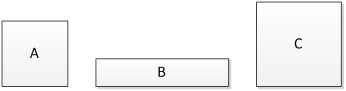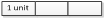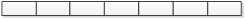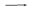### Sample Problem

The area of square A is 49 cm2. The width of rectangle B is the side length of square A. The length of rectangle B is twice the side length of square A. The side length of square C is thrice the width of rectangle B. What is the difference between the area of the square C and the area of rectangle B?

The difference between the area of the square C and the area of rectangle B is cm2.#### Solution

The area of square A is 49 cm2.

Therefore, the side length of square A is 7 cm2.

Width of rectangle BSide length of square A7 units7 cm

1 unit7 cm ÷ 7 = 1 cm

Width of rectangle B ：3 units = 3 × 1 cm = 3 cm

Length of rectangle B = 2 × 7 cm = 14 cm

Area of rectangle B = 3 cm × 14 cm = 42 cm2.

Side length of square C = 3 × 3 cm = 9 cm

Area of square C = 9 cm × 9 cm = 81 cm2.

81 cm2 - 42 cm2 = 39 cm2.

The difference between the area of the square C and the area of rectangle B is 39 cm2.